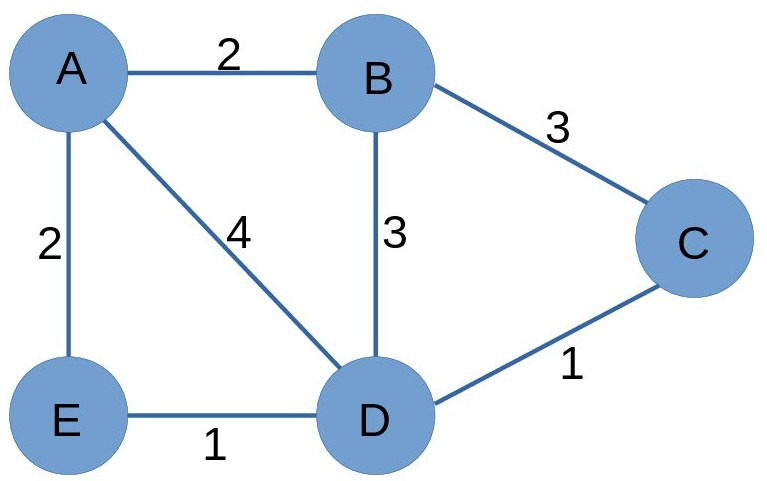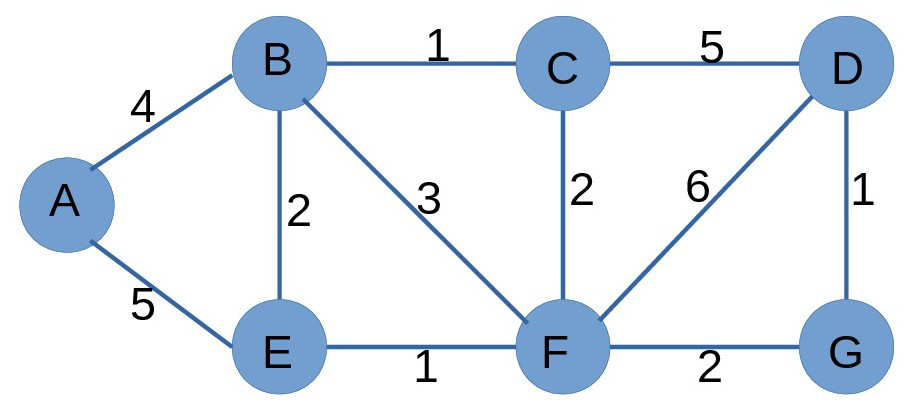Java Program to Optimize Wire Length in Electrical Circuit

• Difficulty Level : Expert
• Last Updated : 22 Jul, 2021

Given n electric components and m electric wires to connect them where each wire has a length. Find the optimized wire length between the two components.

ExampleInput :  Source = A

Destination = C

Output: 4

Explanation: There are five different paths from node A to node C i.e., A->B->C, A->B->D->C, A->D->C, A->E->D->C, A->D->B->C.  But the path with smallest or optimized length is A->E->D->C with length as 4.

Approach

Given n components and m wires constitute an undirected weighted graph. The task is to calculate the optimized length between two components i.e., the minimum length between the two components. The problem is an application of Dijkstra’s algorithm. Since the source is available, the shortest length from the source to all nodes can be calculated using Dijkstra’s algorithm. This will give the shortest possible length between the given node source and all other nodes as an array. Now, the shortest length can be given from the source to destination using this array. Let’s understand this with the help of an example.

Example :Input:    Source = A

Destination = D

Output: 9

Explanation: Use Dijkstra’s algorithm to calculate shortest length from source A to all other nodes.

Vertex        Distance from source

A                   0

B                   4

C                   5

D                   9

E                   5

F                   6

G                   8

The shortest length can be found for any component from source A. Final answer will be the shortest length from A to D i.e., 9.

Below is the implementation of the above approach: